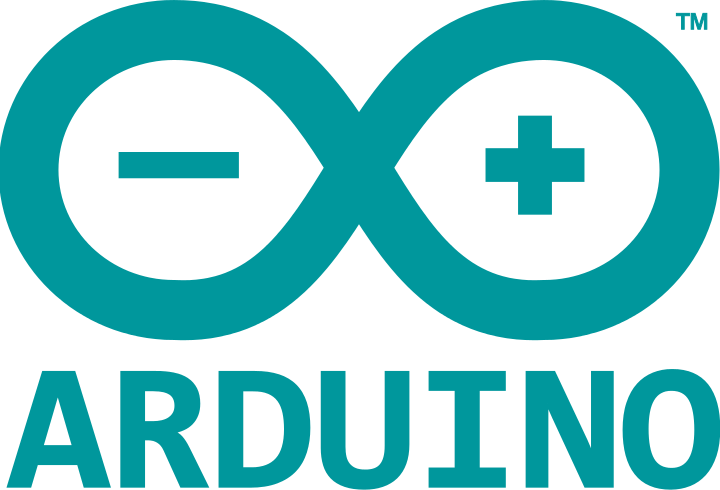# Basics of Electronic Data TransferA picture is worth 1000 words, so they say, (1024 words if you work with computers) so I’ll post some pictures …

I set up my Uno to send “Fab” at 9600 baud and captured the results on a logic analyzer.The parts shaded in red are the “idle” period between bytes.

From the above graphic note that the Tx (transmit) data line is normally high (1) until it drops low to indicate the start of a character (byte). This is the start bit. Then the 8 data bits (indicated by white dots) appear at the baud rate (9600 samples per second). After that the line is brought high again. This is the stop bit (the red part). Then we see the start bit for the next character, and so on. The “stop” portion can be indefinitely long, however it has to be at least one bit length.

More detail for the first character (the letter “F” or 0x46 or 0b01000110) can be seen here:• A – no data (Tx is high)
• B – The “start bit”. The line is taken low to tell the receiver that a character (byte) is commencing to be sent. The receiver waits for one and a half clock times before sampling the line.
• C – First character arrives (the letter “F” or 0x46 or 0b01000110). There is no clock bit as such, the incoming data is simply sampled at the baud (transmission) rate. In contrast to SPI communication the data arrives least-significant bit first (in case you are not sending 8 bits per byte). Thus we see 01100010 (rather than 01000110).
• D – The stop bit. This is always high, to ensure that we can distinguish between the end of this byte, and the start of the next one. Since the start bit is a zero, and the stop bit is a one, there is always a clear transition from one byte to the next.
• E – The start bit for the next character.

You can see from the logic analyzer capture that `T1 - T2` is 0.1041667 ms, and as it happens that is 1/9600:

``1 / 9600 = 0.00010416666 seconds``

Thus the rate of 9600 gives you the number of bits per second and the inverse is the time interval between bits.

## Other considerations

• Serial comms is not self-clocked (unlike SPI or I2C, and others) hence both sender and receiver have to agree on a clock rate.
• The clock rate is not exact on the Arduino, because the hardware has to divide the system clock down to get a serial clock, and the division is not always exact. There is almost always an error, the amount is given in the datasheet (figures quoted for a 16 MHz system clock, such as on the Uno):• You can vary the number of data bits, you don’t have to send 8 of them, in fact you can send 5 to 9 bits.
• There can optionally be a parity bit sent after the data bits.
• If you specify “odd” parity, the parity bit is set in such a way that the total number of 1-bits is odd.
• If you specify “even” parity, the parity bit is set in such a way that the total number of 1-bits is even.
• If you specify no parity, the parity bit is omitted.

This can help the receiver detect if the data arrived correctly or not.

• The parity bit is sent before the stop bit.
• In the case of 9 data bits (as used in the SeaTalk protocol) the parity bit is re-purposed as a 9th data bit. Therefore you can’t have both 9 data bits and a parity bit.
• You can also have two stop bits. This basically just lengthens the time between bytes. In the “olden days” this was so that slow electromechanical equipment could process the previous byte (eg. to print it).

### Possible corruption

If you start listening to serial data in the middle of a stream, it is quite possible that a 0-bit in the middle of the stream will be interpreted as a start bit, and then the receiver will interpret everything after that incorrectly.

The only real way to recover from this is to have a large enough gap, from time to time, (eg. 10 bits long) that this cannot happen.

### Inverted logic

The bits shown here (logic-level) are not inverted. That is, a 1-bit is HIGH and a 0-bit is LOW. If you have RS232 equipment that will probably be sending something like -12 V for a 1-bit, and +12 V for a 0-bit. This is inverted because a one is less than a zero, voltage-wise.

If you have such devices you need to do voltage conversion and logic inversion. Chips like the MAX232 will do both for you. They can also provide the -12 V needed to drive such equipment by generating it internally with the help of a few user-supplied capacitors.

### Speed rule-of-thumb

Since, with one start bit, 8 data bits, and one stop bit we have a total of 10 bits, as a quick rule of thumb, you can calculate the number of bytes you can transmit in a second by dividing the bit rate by 10.

Eg. At 9600 BPS you can send 960 bytes per second.

Code to reproduce:

``````void setup()
{
Serial.begin(9600);
Serial.print("Fab");
}

void loop ()
{
}``````

#### How to setup Zoho email in Lucee

This site uses Akismet to reduce spam. Learn how your comment data is processed.